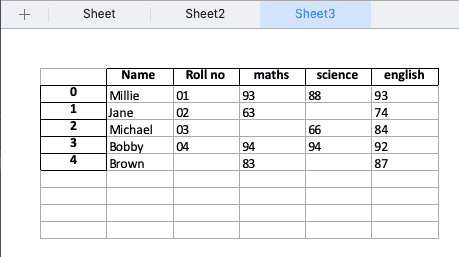# How to Use Pandas.ExcelWriter Method in Python

Pandas.ExcelWriter() is a class for writing DataFrame objects into excel sheets. The ExcelWriter() can be used to write text, number, strings, formulas. It can work on multiple worksheets also. Python Pandas is a data analysis library. It can read, filter, and re-arrange small and large data sets and output them in a range of formats, including Excel. The ExcelWriter() is defined under the Pandas library.

For this example, you had installed the Numpy and Pandas library on your machine.

### Syntax

```pandas.ExcelWriter(path, engine= None, date_format=None, datetime_format=None, mode=’w’,**engine_krawgs)
```

### Parameters

All the parameters are set to default values.

Pandas.ExcelWriter() function has five parameters.

1. path: It is of string type, which indicates the path to the xls or xlsx file.
2. engine: It is also of string type and is entirely optional. It is the engine to use for writing.
3. date_format: It is also of string type and has a default value of None. It formats the string for dates written into Excel Files.
4. datetime_format: It is also of string type and has a default value of None. It formats the string for datetime objects written into Excel Files.
5. Mode: It is the mode of a file to use that is to write or append. Its default value is to write, which is ‘w‘.

### Return Value

It exports the data into an Excel file.

### Example program on Pandas ExcelWriter()

You have to install and import xlsxwriter module. If you are running a Jupyter notebook, then you won’t need it; otherwise, you have to install it. You can find the installation guide here.

Write a program to show the working of the ExcelWriter() in Python.

```import pandas as pd
import numpy as np
import xlsxwriter

# Creating dataset using dictionary
data_set = {
'Name': ['Rohit', 'Arun', 'Sohit', 'Arun', 'Shubh'],
'Roll no': ['01', '02', '03', '04', np.nan],
'maths': ['93', '63', np.nan, '94', '83'],
'science': ['88', np.nan, '66', '94', np.nan],
'english': ['93', '74', '84', '92', '87']}

# Converting into dataframe
df = pd.DataFrame(data_set)

# Writing the data into the excel sheet
writer_obj = pd.ExcelWriter('Write.xlsx',
engine='xlsxwriter')

df.to_excel(writer_obj, sheet_name='Sheet')

writer_obj.save()
print('Please check out the Write.xlsx file.')
```

#### Output

`Please check out the Write.xlsx file.`

The content of the excel file is following.In the above code, we created a DataFrame that stored the data of students. Then we created a writer object to write the data of the DataFrame into an excel sheet, and after writing the data in the sheet, we saved the sheet. Some values in the above excel sheet are empty because, in the DataFrame, those values are np.nan. To check the data of the DataFrame, do check the excel sheet.

## Pandas DataFrame to_excel()

Pandas DataFrame to_excel() function writes an object to the Excel sheet. We have used the to_excel() function in the above example because ExcelWriter() method returns the writer object and then we use the DataFrame.to_excel() method to export it into an Excel file.

To write a single object to the Excel .xlsx file, it is only necessary to specify a target file name. To write to multiple sheets, it is required to create the ExcelWriter object with a target file name, and specify the sheet in the file to write to.

Multiple sheets may be written to by specifying the unique sheet_name. With all data written to a file, it is necessary to save the changes. Note that creating the ExcelWriter object with a file name that already exists and will result in the contents of an existing file being erased.

We can also write the above example using Python with statement.

```import pandas as pd
import numpy as np
import xlsxwriter

# Creating dataset using dictionary
data_set = {
'Name': ['Rohit', 'Arun', 'Sohit', 'Arun', 'Shubh'],
'Roll no': ['01', '02', '03', '04', np.nan],
'maths': ['93', '63', np.nan, '94', '83'],
'science': ['88', np.nan, '66', '94', np.nan],
'english': ['93', '74', '84', '92', '87']}

# Converting into dataframe
df = pd.DataFrame(data_set)

with pd.ExcelWriter('WriteWith.xlsx', engine='xlsxwriter') as writer:
df.to_excel(writer, sheet_name='Sheet')

print('Please check out the WriteWith.xlsx file.')
```

#### Output

`Please check out the WriteWith.xlsx file.`

You can check out the WriteWith.xlsx file and see its content. It will be the same as the Write.xlsx file.

## Writing multiple DataFrames to multiple sheets.

In the above example, we have seen only a single sheet for a single DataFrame. We can write multiple Frames with multiple sheets using Pandas.ExcelWriter.

Let’s write an example in which we will create three DataFrames and save those DataFrames in multiplesheet.xlsx file with three different sheets.

```import pandas as pd
import numpy as np
import xlsxwriter

# Creating dataset using dictionary
data_set = {
'Name': ['Rohit', 'Arun', 'Sohit', 'Arun', 'Shubh'],
'Roll no': ['01', '02', '03', '04', np.nan],
'maths': ['93', '63', np.nan, '94', '83'],
'science': ['88', np.nan, '66', '94', np.nan],
'english': ['93', '74', '84', '92', '87']}

data_set2 = {
'Name': ['Ankit', 'Krunal', 'Rushabh', 'Dhaval', 'Nehal'],
'Roll no': ['01', '02', '03', '04', np.nan],
'maths': ['93', '63', np.nan, '94', '83'],
'science': ['88', np.nan, '66', '94', np.nan],
'english': ['93', '74', '84', '92', '87']}

data_set3 = {
'Name': ['Millie', 'Jane', 'Michael', 'Bobby', 'Brown'],
'Roll no': ['01', '02', '03', '04', np.nan],
'maths': ['93', '63', np.nan, '94', '83'],
'science': ['88', np.nan, '66', '94', np.nan],
'english': ['93', '74', '84', '92', '87']}

# Converting into dataframe
df = pd.DataFrame(data_set)
df2 = pd.DataFrame(data_set2)
df3 = pd.DataFrame(data_set3)

with pd.ExcelWriter('multiplesheet.xlsx', engine='xlsxwriter') as writer:
df.to_excel(writer, sheet_name='Sheet')
df2.to_excel(writer, sheet_name='Sheet2')
df3.to_excel(writer, sheet_name='Sheet3')

print('Please check out the multiplesheet.xlsx file.')
```

#### OutputYou can see that there are three sheets, and each sheet has different Name columns.

The to_excel() function accepts sheet_name as a parameter, and here we can pass the three different sheet names and that DataFrame is saved in the respective sheets.

## Conclusion

If you want to export Pandas DataFrame to Excel files, then ExcelWriter() class is all you need. The ExcelWrite() class gives the writer object, and then we can use the to_excel() function to export the DataFrame to Excel file. That’s it for the Pandas.ExcelWriter class.